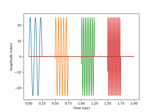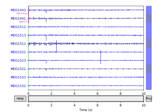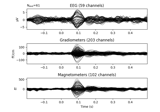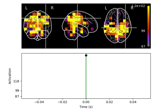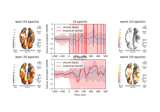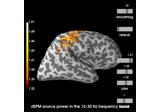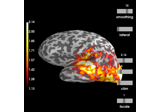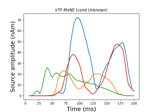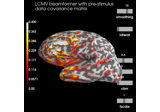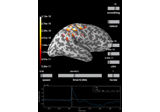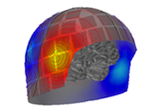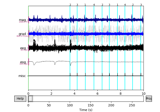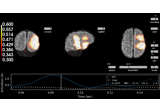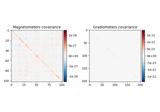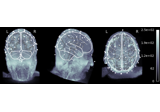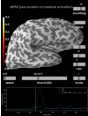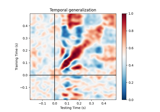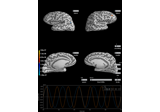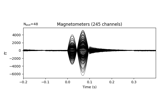# mne.compute_covariance¶

mne.compute_covariance(epochs, keep_sample_mean=True, tmin=None, tmax=None, projs=None, method='empirical', method_params=None, cv=3, scalings=None, n_jobs=1, return_estimators=False, on_mismatch='raise', rank=None, verbose=None)[source]

Estimate noise covariance matrix from epochs.

The noise covariance is typically estimated on pre-stimulus periods when the stimulus onset is defined from events.

If the covariance is computed for multiple event types (events with different IDs), the following two options can be used and combined:

1. either an Epochs object for each event type is created and a list of Epochs is passed to this function.

2. an Epochs object is created for multiple events and passed to this function.

Note

To estimate the noise covariance from non-epoched raw data, such as an empty-room recording, use mne.compute_raw_covariance() instead.

Parameters
epochsinstance of Epochs, or list of Epochs

The epochs.

keep_sample_meanbool (default True)

If False, the average response over epochs is computed for each event type and subtracted during the covariance computation. This is useful if the evoked response from a previous stimulus extends into the baseline period of the next. Note. This option is only implemented for method=’empirical’.

tmin

Start time for baseline. If None start at first sample.

tmax

End time for baseline. If None end at last sample.

projs

List of projectors to use in covariance calculation, or None to indicate that the projectors from the epochs should be inherited. If None, then projectors from all epochs must match.

methodstr | list | None (default ‘empirical’)

The method used for covariance estimation. If ‘empirical’ (default), the sample covariance will be computed. A list can be passed to perform estimates using multiple methods. If ‘auto’ or a list of methods, the best estimator will be determined based on log-likelihood and cross-validation on unseen data as described in . Valid methods are ‘empirical’, ‘diagonal_fixed’, ‘shrunk’, ‘oas’, ‘ledoit_wolf’, ‘factor_analysis’, ‘shrinkage’, and ‘pca’ (see Notes). If 'auto', it expands to:

['shrunk', 'diagonal_fixed', 'empirical', 'factor_analysis']


'factor_analysis' is removed when rank is not ‘full’. The 'auto' mode is not recommended if there are many segments of data, since computation can take a long time.

New in version 0.9.0.

method_params

Additional parameters to the estimation procedure. Only considered if method is not None. Keys must correspond to the value(s) of method. If None (default), expands to the following (with the addition of {'store_precision': False, 'assume_centered': True} for all methods except 'factor_analysis' and 'pca'):

{'diagonal_fixed': {'grad': 0.1, 'mag': 0.1, 'eeg': 0.1, ...},
'shrinkage': {'shrikage': 0.1},
'shrunk': {'shrinkage': np.logspace(-4, 0, 30)},
'pca': {'iter_n_components': None},
'factor_analysis': {'iter_n_components': None}}

cvint | sklearn.model_selection object (default 3)

The cross validation method. Defaults to 3, which will internally trigger by default sklearn.model_selection.KFold with 3 splits.

scalings

Defaults to dict(mag=1e15, grad=1e13, eeg=1e6). These defaults will scale data to roughly the same order of magnitude.

n_jobsint

The number of jobs to run in parallel (default 1). Requires the joblib package.

return_estimatorsbool (default False)

Whether to return all estimators or the best. Only considered if method equals ‘auto’ or is a list of str. Defaults to False.

on_mismatchstr

What to do when the MEG<->Head transformations do not match between epochs. If “raise” (default) an error is raised, if “warn” then a warning is emitted, if “ignore” then nothing is printed. Having mismatched transforms can in some cases lead to unexpected or unstable results in covariance calculation, e.g. when data have been processed with Maxwell filtering but not transformed to the same head position.

rankNone | dict | ‘info’ | ‘full’

This controls the rank computation that can be read from the measurement info or estimated from the data. See Notes of mne.compute_rank() for details.The default is None.

New in version 0.17.

New in version 0.18: Support for ‘info’ mode.

verbose

If not None, override default verbose level (see mne.verbose() and Logging documentation for more). If used, it should be passed as a keyword-argument only.

Returns
covinstance of Covariance | list

The computed covariance. If method equals ‘auto’ or is a list of str and return_estimators equals True, a list of covariance estimators is returned (sorted by log-likelihood, from high to low, i.e. from best to worst).

compute_raw_covariance

Estimate noise covariance from raw data, such as empty-room recordings.

Notes

Baseline correction or sufficient high-passing should be used when creating the Epochs to ensure that the data are zero mean, otherwise the computed covariance matrix will be inaccurate.

Valid method strings are:

• 'empirical'

The empirical or sample covariance (default)

• 'diagonal_fixed'

A diagonal regularization based on channel types as in mne.cov.regularize().

• 'shrinkage'

Fixed shrinkage.

New in version 0.16.

• 'ledoit_wolf'

The Ledoit-Wolf estimator, which uses an empirical formula for the optimal shrinkage value .

• 'oas'

The OAS estimator , which uses a different empricial formula for the optimal shrinkage value.

New in version 0.16.

• 'shrunk'

Like ‘ledoit_wolf’, but with cross-validation for optimal alpha.

• 'pca'

Probabilistic PCA with low rank .

• 'factor_analysis'

Factor analysis with low rank .

'ledoit_wolf' and 'pca' are similar to 'shrunk' and 'factor_analysis', respectively, except that they use cross validation (which is useful when samples are correlated, which is often the case for M/EEG data). The former two are not included in the 'auto' mode to avoid redundancy.

For multiple event types, it is also possible to create a single Epochs object with events obtained using mne.merge_events(). However, the resulting covariance matrix will only be correct if keep_sample_mean is True.

The covariance can be unstable if the number of samples is small. In that case it is common to regularize the covariance estimate. The method parameter allows to regularize the covariance in an automated way. It also allows to select between different alternative estimation algorithms which themselves achieve regularization. Details are described in .

References

1(1,2)

Engemann D. and Gramfort A. (2015) Automated model selection in covariance estimation and spatial whitening of MEG and EEG signals, vol. 108, 328-342, NeuroImage.

2

Ledoit, O., Wolf, M., (2004). A well-conditioned estimator for large-dimensional covariance matrices. Journal of Multivariate Analysis 88 (2), 365 - 411.

3

Tipping, M. E., Bishop, C. M., (1999). Probabilistic principal component analysis. Journal of the Royal Statistical Society: Series B (Statistical Methodology) 61 (3), 611 - 622.

4

Barber, D., (2012). Bayesian reasoning and machine learning. Cambridge University Press., Algorithm 21.1

5

Chen et al. (2010). Shrinkage Algorithms for MMSE Covariance Estimation. IEEE Trans. on Sign. Proc., Volume 58, Issue 10, October 2010.

## Examples using mne.compute_covariance¶